Balbharti 12th Maharashtra State Board Maths Solutions Book Pdf Chapter 3 Trigonometric Functions Ex 3.3 Questions and Answers.

## Maharashtra State Board 12th Maths Solutions Chapter 3 Trigonometric Functions Ex 3.3

Question 1.
Find the principal values of the following :
(i) sin-1$$\left(\frac{1}{2}\right)$$
Solution:
The principal value branch of sin-1x is $$\left[-\frac{\pi}{2}, \frac{\pi}{2}\right]$$.
Let sin-1$$\left(\frac{1}{2}\right)$$ = ∝, where $$\frac{-\pi}{2}$$ ≤ ∝ ≤ $$\frac{\pi}{2}$$
∴ sin∝ = $$\frac{1}{2}$$ = sin$$\frac{\pi}{6}$$
∴ ∝ = $$\frac{\pi}{6}$$ …[∵ – $$\frac{\pi}{2}$$ ≤ $$\frac{\pi}{6}$$ ≤ $$\frac{\pi}{2}$$]
∴ the principal value of sin-1$$\left(\frac{1}{2}\right)$$ is $$\frac{\pi}{6}$$.

(ii) cosec-1(2)
Solution:
The principal value branch of cosec-1x is $$\left[-\frac{\pi}{2}, \frac{\pi}{2}\right]$$ – {0}.
Let cosec-1(2) = ∝, where $$\frac{-\pi}{2}$$ ≤ ∝ ≤ $$\frac{\pi}{2}$$, ∝ ≠ 0
∴ cosec-1 ∝ = 2 = cosec$$\frac{\pi}{6}$$
∴ ∝ = $$\frac{\pi}{6}$$ …[∵ –$$\frac{\pi}{2}$$ ≤ $$\frac{\pi}{6}$$ ≤ $$\frac{\pi}{2}$$]
∴ the principal value of cosec-1(2) is $$\frac{\pi}{6}$$.(iii) tan-1(-1)
Solution:
The principal value branch of tan-1x is $$\left(-\frac{\pi}{2}, \frac{\pi}{2}\right)$$
Let tan-1(-1) = ∝, where $$\frac{-\pi}{2}$$ < ∝ < $$\frac{\pi}{2}$$
∴ tan∝ = -1 = -tan$$\frac{\pi}{4}$$
∴ tan∝ = tan$$\left(-\frac{\pi}{4}\right)$$ …[∵ tan(-θ) = -tanθ]
∴ ∝ = –$$\frac{\pi}{4}$$ …[∵ –$$\frac{\pi}{2}$$ < $$\frac{-\pi}{4}$$ < $$\frac{\pi}{2}$$]
∴ the principal value of tan-1(-1) is –$$\frac{\pi}{4}$$.(iv) tan-1(-$$\sqrt {3}$$)
Solution:
The principal value branch of tan-1x is $$\left(-\frac{\pi}{2}, \frac{\pi}{2}\right)$$.
Let tan-1(-$$\sqrt {3}$$) = ∝, where $$\frac{-\pi}{2}$$ < ∝ < $$\frac{\pi}{2}$$
∴ tan∝ = –$$\sqrt {3}$$ = -tan$$\frac{\pi}{3}$$
∴ tan∝ = tan$$\left(-\frac{\pi}{3}\right)$$ …[∵ tan(-θ) = -tanθ]
∴ ∝ = –$$\frac{\pi}{3}$$ …[∵ –$$\frac{\pi}{2}$$ < $$\frac{-\pi}{3}$$ < $$\frac{\pi}{2}$$]
∴ the principal value of tan-1(-$$\sqrt {3}$$) is –$$\frac{\pi}{3}$$.

(v) sin-1 $$\left(\frac{1}{\sqrt{2}}\right)$$
Solution:
The principal value branch of sin-1x is $$\left[-\frac{\pi}{2}, \frac{\pi}{2}\right]$$.
Let sin-1 $$\left(\frac{1}{\sqrt{2}}\right)$$ = ∝, where $$\frac{-\pi}{2}$$ < ∝ < $$\frac{\pi}{2}$$
∴ sin∝ = $$\left(\frac{1}{\sqrt{2}}\right)$$ = sin$$\frac{\pi}{4}$$
∴ ∝ = $$\frac{\pi}{4}$$ …[∵ –$$\frac{\pi}{2}$$ ≤ $$\frac{\pi}{4}$$ ≤ $$\frac{\pi}{2}$$]
∴ the principal value of sin-1 $$\left(\frac{1}{\sqrt{2}}\right)$$ is $$\frac{\pi}{4}$$.

(vi) cos-1$$\left(-\frac{1}{2}\right)$$
Solution:
The principal value branch of cos-1x is (0, π).
Let cos-1$$\left(-\frac{1}{2}\right)$$ = ∝, where 0 ≤ ∝ ≤ π
∴ cos∝ = $$-\frac{1}{2}$$ = -cos$$\frac{\pi}{3}$$
∴ cos∝ = cos$$\left(\pi-\frac{\pi}{3}\right)$$ …[∵ cos(π – θ) = -cosθ)
∴ cos∝ = cos$$\frac{2 \pi}{3}$$
∴ ∝ = $$\frac{2 \pi}{3}$$ …[∵ 0 ≤ $$\frac{2 \pi}{3}$$ ≤ π]
∴ the principal value of cos-1$$\left(-\frac{1}{2}\right)$$ is $$\frac{2 \pi}{3}$$.Question 2.
Evaluate the following :
(i) tan-1(1) + cos-1$$\left(\frac{1}{2}\right)$$ + sin-1$$\left(\frac{1}{2}\right)$$
Solution: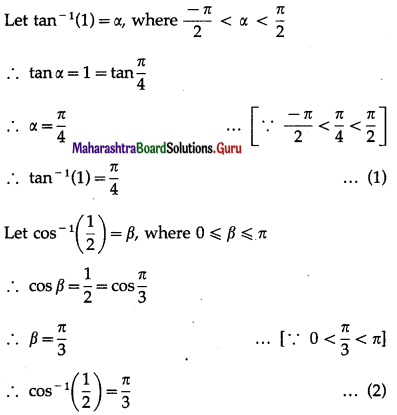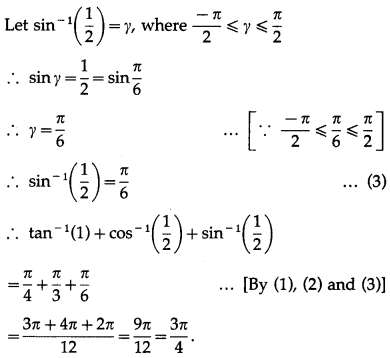(ii) cos-1$$\left(\frac{1}{2}\right)$$ + 2 sin-1$$\left(\frac{1}{2}\right)$$
Solution: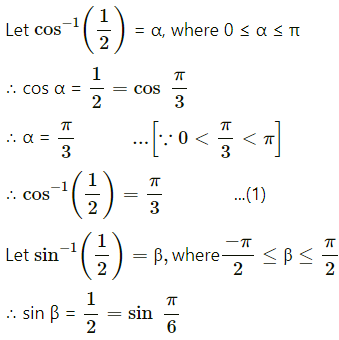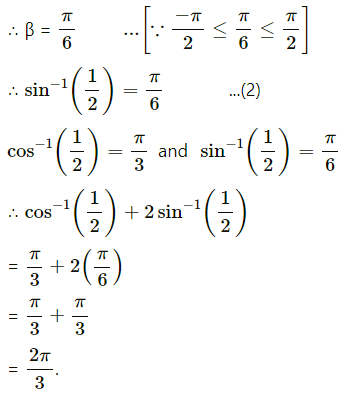(iii) tan-1$$\sqrt {3}$$ – sec-1(-2)
Solution: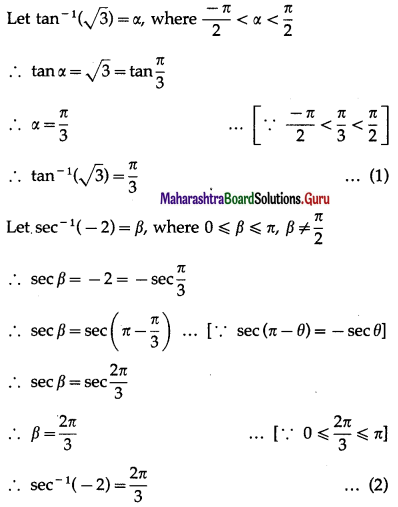∴ tan-1$$\sqrt {3}$$ – sec-1(-2)
= $$\frac{\pi}{3}-\frac{2 \pi}{3}$$ …[By (1) and (2)]
= –$$\frac{\pi}{3}$$.(iv) cosec-1( $$-\sqrt{2}$$) + cot-1($$\sqrt{3}$$)
Solution: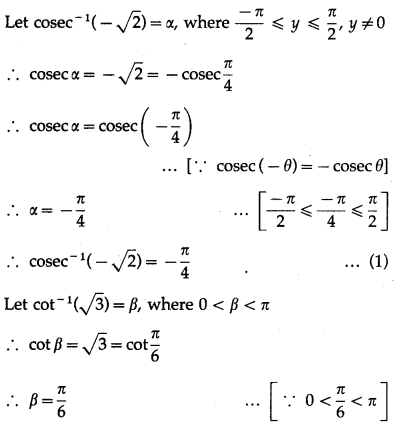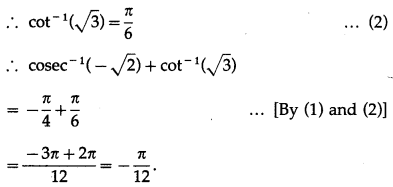Question 3.
Prove the following :
(i) sin-1$$\left(\frac{1}{\sqrt{2}}\right)$$ – 3sin-1$$\left(\frac{\sqrt{3}}{2}\right)$$ = –$$-\frac{3 \pi}{4}$$
Question is modified.
sin-1$$\left(\frac{1}{\sqrt{2}}\right)$$ – 3sin-1$$\left(\frac{\sqrt{3}}{2}\right)$$ = –$$\frac{3 \pi}{4}$$
Solution: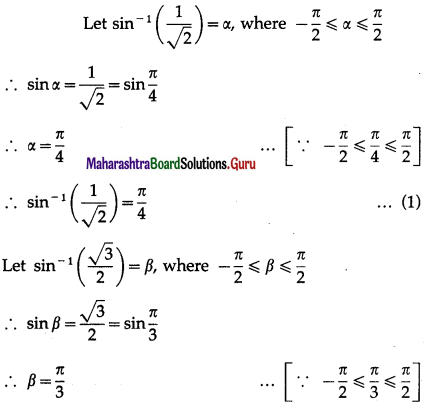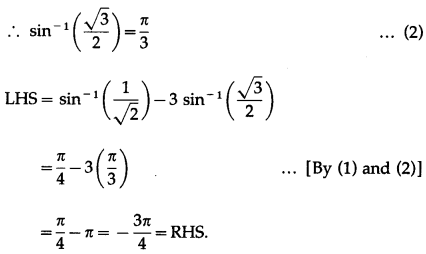(ii) sin-1$$\left(-\frac{1}{2}\right)$$ + cos-1$$\left(-\frac{\sqrt{3}}{2}\right)$$ = cos-1$$\left(-\frac{1}{2}\right)$$
Solution: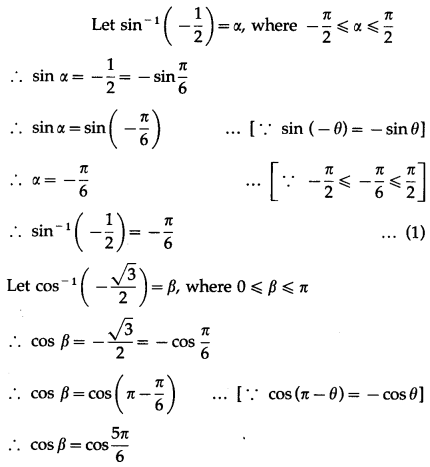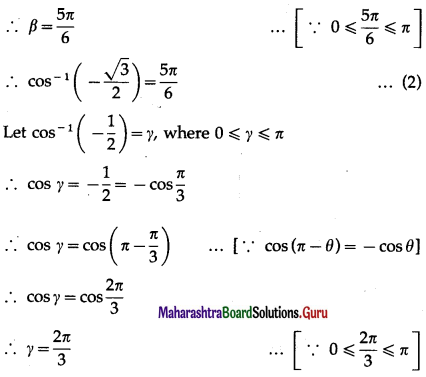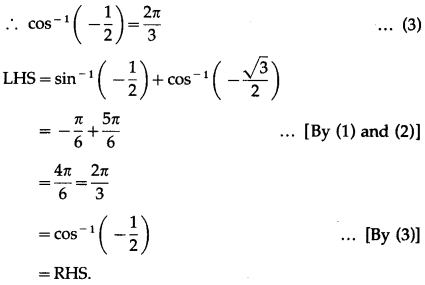(iii) sin-1$$\left(\frac{3}{5}\right)$$ + cos-1$$\left(\frac{12}{13}\right)$$ = sin-1$$\left(\frac{56}{65}\right)$$
Solution: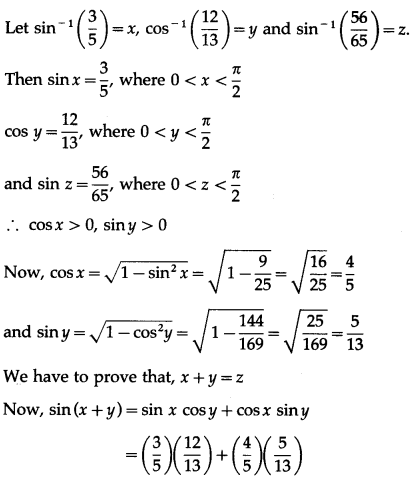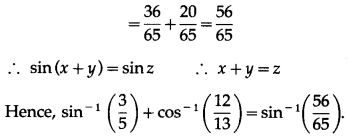(iv) cos-1$$\left(\frac{3}{5}\right)$$ + cos-1$$\left(\frac{4}{5}\right)$$ = $$\frac{\pi}{2}$$
Solution:
Let cos-1$$\left(\frac{3}{5}\right)$$ = x
∴ cosx = $$\left(\frac{3}{5}\right)$$, where 0 < x < $$\frac{\pi}{2}$$ ∴ sinx > 0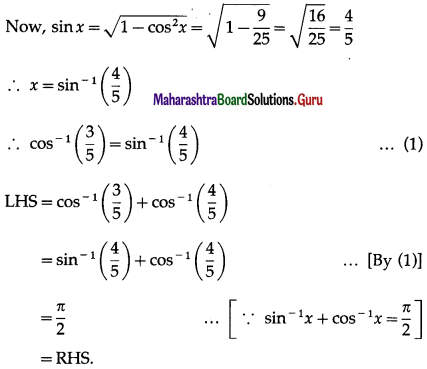(v) tan-1$$\left(\frac{1}{2}\right)$$ + tan-1$$\left(\frac{1}{3}\right)$$ = $$\frac{\pi}{4}$$
Solution:
LHS = tan-1$$\left(\frac{1}{2}\right)$$ + tan-1$$\left(\frac{1}{3}\right)$$
= tan-1$$\left(\frac{\frac{1}{2}+\frac{1}{3}}{1-\frac{1}{2} \times \frac{1}{3}}\right)$$
= tan-1$$\left(\frac{3+2}{6-1}\right)$$ = tan-1(1)
= tan-1$$\left(\tan \frac{\pi}{4}\right)$$ = $$\frac{\pi}{4}$$
= RHS.(vi) 2 tan-1$$\left(\frac{1}{3}\right)$$ = tan-1$$\left(\frac{3}{4}\right)$$
Solution: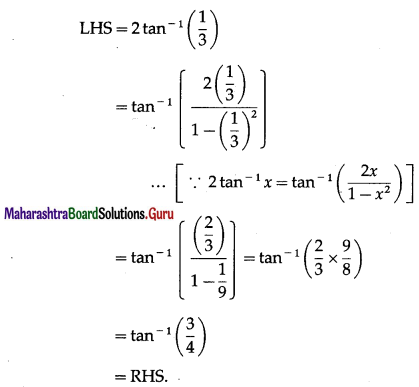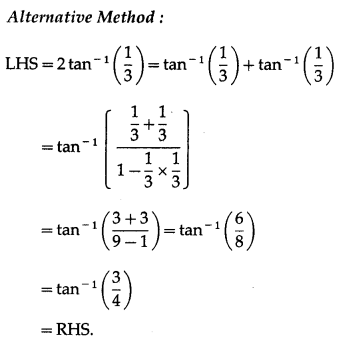(vii) tan-1$$\left[\frac{\cos \theta+\sin \theta}{\cos \theta-\sin \theta}\right]$$ = $$\frac{\pi}{4}$$ + θ if θ ∈ $$\left(-\frac{\pi}{4}, \frac{\pi}{4}\right)$$
Solution: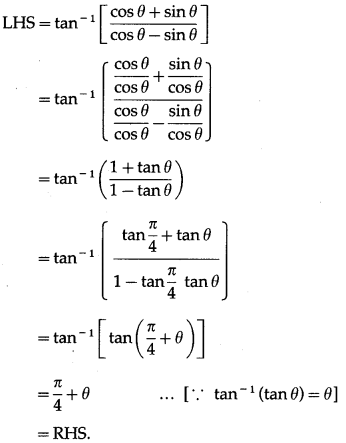(viii) tan-1$$\sqrt{\frac{1-\cos \theta}{1+\cos \theta}}=\frac{\theta}{2}$$, if θ ∈ (0, π)
Solution: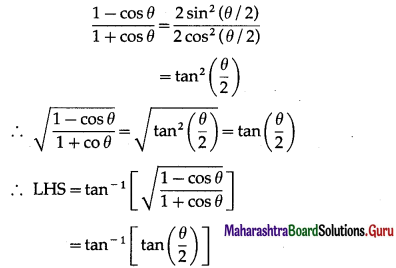= $$\frac{\theta}{2}$$ …[∵ tan-1(tanθ) = θ]
= RHS.